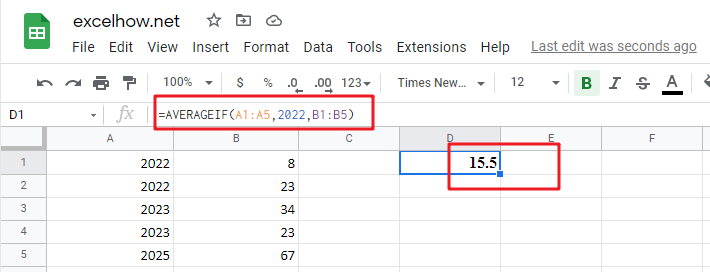# ExcelHow

This post will guide you how to use Google Sheets AVERAGEIF function with syntax and examples in Google Spreadsheet.

## Description

The Google Sheets AVERAGEIF function returns the average of all numbers in a range of cells that meet a given criteria.

The AVERAGEIF function is a build-in function in Google Sheets and it is categorized as a Statistical Function.

## Syntax

The syntax of the AVERAGEIF function is as below:

`= AVERAGEIF (range, criteria, [average_range])`

Where the AVERAGEIF function arguments are:

• `Range `-This is a required argument.  The range of cells that you want to apply the criteria to average
• `Criteria `– This is a required argument.  The criteria used to define which cells are averaged
• `Average_range`This is an optional argument.  The average range is actually to average. If it is omitted, the function will use Range values to average.

## Google Sheets AVERAGEIF Function Example

The below examples will show you how to use Google Sheets AVERAGEIF Function to return the average of all numbers in a range of cells based on a given criteria.

#1 To get the average of all numbers in average range B1:B5 and meet the criteria that equal to “2022” in range A1:A5, and the, just using the following Google Sheets formula:

`=AVERAGEIF(A1:A5,2022,B1:B5)`